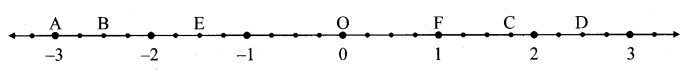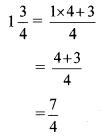# Maharashtra Board Class 8 Maths Solutions Chapter 1 Rational and Irrational Numbers Practice Set 1.1

## Maharashtra State Board Class 8 Maths Solutions Chapter 1 Rational and Irrational Numbers Practice Set 1.1

Question 1.
Show the following numbers on a number line. Draw a separate number line for each example.
i. $$\frac{3}{2}, \frac{5}{2},-\frac{3}{2}$$
ii. $$\frac{7}{5}, \frac{-2}{5}, \frac{-4}{5}$$
iii. $$\frac{-5}{8}, \frac{11}{8}$$
iv. $$\frac{13}{10}, \frac{-17}{10}$$
Solution:
i. $$\frac{3}{2}, \frac{5}{2},-\frac{3}{2}$$Here, the denominator of each fraction is 2.
∴ Each unit will be divided into 2 equal parts.

ii. $$\frac{7}{5}, \frac{-2}{5}, \frac{-4}{5}$$Here, the denominator of each fraction is 5.
∴ Each unit will be divided into 5 equal parts.

iii. $$\frac{-5}{8}, \frac{11}{8}$$Here, the denominator of each fraction is 8.
∴ Each unit will be divided into 8 equal parts.

iv. $$\frac{13}{10}, \frac{-17}{10}$$Here, the denominator of each fraction is 10.
∴ Each unit will be divided into 10 equal parts.

Question 2.
Observe the number line and answer the questions.i. Which number is indicated by point B?
ii. Which point indicates the number $$1\frac { 3 }{ 4 }$$ ?
iii. State whether the statement, ‘the point D denotes the number $$\frac { 5 }{ 2 }$$ is true or false.
Solution:
Here, each emit is divided into 4 equal parts.
i. Point B is marked on the 10th equal part on the left side of O.
∴ The number indicated by point B is $$\frac { -10 }{ 4 }$$.

ii.Point C is marked on the 7th equal part on the right side of O.
∴ The number $$1\frac { 3 }{ 4 }$$ is indicated by point C.

iii. True
Point D is marked on the 10th equal part on the right side of O.
∴ D denotes the number $$\frac{10}{4}=\frac{5 \times 2}{2 \times 2}=\frac{5}{2}$$

Scroll to Top